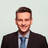Having Trouble with print to pdf

Dear Ninox Team,

i have the following code which basically takes multiple lists of material and puts it into one. The function also counts and deletes double entries.

It works just like i want it, but if i open the print to pdf section and use this function to print the list, it just hangs with the moving circle. I have to refresh the page to get access to the datebase again.

Do you have any idea how to solve this issue?

let cnt_Nodes := 0;
let cnt_PBoxes := 0;
let cnt_EBoxes := 0;
let cnt_Harddisks := 0;
let cnt_HDDs := 0;
let time_start := number(now());
let stop := false;
let WordCntString := concat(Aufgaben.'FSA Aufgaben'.Material);
while length(WordCntString) > 5 and contains(WordCntString, "Knoten") and stop = false do
WordCntString := substring(WordCntString, index(WordCntString, "Knoten") + 6, length(WordCntString));
cnt_Nodes := cnt_Nodes + 1;
let time_end := number(now());
if time_end - time_start > 2000 then
stop := true
end
end
;
let WordCntString := concat(Aufgaben.'FSA Aufgaben'.Material);
while length(WordCntString) > 5 and contains(WordCntString, "Pumpenbox") and stop = false do
WordCntString := substring(WordCntString, index(WordCntString, "Pumpenbox") + 9, length(WordCntString));
cnt_PBoxes := cnt_PBoxes + 1;
let time_end := number(now());
if time_end - time_start > 2000 then
stop := true
end
end
;
let WordCntString := concat(Aufgaben.'FSA Aufgaben'.Material);
while length(WordCntString) > 5 and contains(WordCntString, "Elektrobox") and stop = false do
WordCntString := substring(WordCntString, index(WordCntString, "Elektrobox") + 10, length(WordCntString));
cnt_EBoxes := cnt_EBoxes + 1;
let time_end := number(now());
if time_end - time_start > 2000 then
stop := true
end
end
;
let WordCntString := concat(Aufgaben.'FSA Aufgaben'.Material);
while length(WordCntString) > 5 and contains(WordCntString, "Festplatte") and stop = false do
WordCntString := substring(WordCntString, index(WordCntString, "Festplatte") + 10, length(WordCntString));
cnt_Harddisks := cnt_Harddisks + 1;
let time_end := number(now());
if time_end - time_start > 2000 then
stop := true
end
end
;
let WordCntString := concat(Aufgaben.'FSA Aufgaben'.Material);
while length(WordCntString) > 2 and contains(WordCntString, "HDD") and stop = false do
WordCntString := substring(WordCntString, index(WordCntString, "HDD") + 3, length(WordCntString));
cnt_HDDs := cnt_HDDs + 1;
let time_end := number(now());
if time_end - time_start > 2000 then
stop := true
end
end
;
let MaterialString := concat(Aufgaben.'FSA Aufgaben'.Material);
MaterialString := replace(MaterialString, ", ", "");
MaterialString := replace(MaterialString, "- ", "%\$- ");
MaterialString := replacex(MaterialString, "\n", "g", "");
let MaterialStringArray := split(MaterialString, "%\$");
MaterialStringArray := unique(MaterialStringArray);
let AuxString := concat(MaterialStringArray);
if cnt_Nodes > 1 then
let AuxString1 := substr(AuxString, 0, index(AuxString, "Knoten") + 6);
let AuxString2 := substr(AuxString, index(AuxString, "Knoten") + 6, length(AuxString));
AuxString := AuxString1 + " (" + cnt_Nodes + ")" + AuxString2
end;
if cnt_PBoxes > 1 then
let AuxString1 := substr(AuxString, 0, index(AuxString, "Pumpenbox") + 9);
let AuxString2 := substr(AuxString, index(AuxString, "Pumpenbox") + 9, length(AuxString));
AuxString := AuxString1 + "en (" + cnt_PBoxes + ")" + AuxString2
end;
if cnt_EBoxes > 1 then
let AuxString1 := substr(AuxString, 0, index(AuxString, "Elektrobox") + 10);
let AuxString2 := substr(AuxString, index(AuxString, "Elektrobox") + 10, length(AuxString));
AuxString := AuxString1 + "en (" + cnt_EBoxes + ")" + AuxString2
end;
if cnt_Harddisks > 1 then
let AuxString1 := substr(AuxString, 0, index(AuxString, "Festplatte") + 10);
let AuxString2 := substr(AuxString, index(AuxString, "Festplatte") + 10, length(AuxString));
AuxString := AuxString1 + "n (" + cnt_Harddisks + ")" + AuxString2
end;
if cnt_HDDs > 1 then
let AuxString1 := substr(AuxString, 0, index(AuxString, "HDD") + 3);
let AuxString2 := substr(AuxString, index(AuxString, "HDD") + 3, length(AuxString));
AuxString := AuxString1 + "s (" + cnt_HDDs + ")" + AuxString2
end;
replace(replace(AuxString, ", - ", "
[_] "), "- ", "[_] ")Hi we would have to take a closer look. Would you be up for a screen sharing session?

https://calendly.com/alexander-koenig/15min

Best regards,

Alex

Dear Alex,

thanks for your support. I think i figured it out by myself. I used to create this string "concat(Aufgaben.'FSA Aufgaben'.Material);" a way to often. I solved it by do it only once and store it into a variable. So i was able to use the variable instead of creating the string again and again.

You can consider it as solved

Kind regards